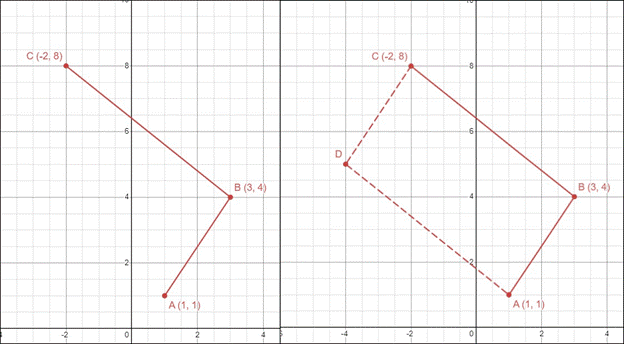# CAT 2022 Question Paper | Quant Slot 1

###### CAT Previous Year Paper | CAT Quant Questions | Question 1

CAT 2022 Quant was dominated by Arithmetic followed by Algebra. In Arithmetic, the questions were dominated by topics like Speed-time-distance, Mixture and Alligations. This year, there was a surprise. The questions from Geometry were relatively on the lower side as compared to the previous years. There were 8 TITA Qs this year. Overall this section was at a medium level of difficulty.

Question 1 : Let ABCD be a parallelogram such that the coordinates of its three vertices A, B, C are (1, 1), (3, 4) and (-2, 8), respectively. Then, the coordinates of the vertex D are

1. (-4, 5)
2. (4, 5)
3. (-3, 4)
4. (0, 11)

## Best CAT Coaching in Chennai

#### CAT Coaching in Chennai - CAT 2022Limited Seats Available - Register Now!

Let us locate the three given points on a 2D graph.
The fourth corner D of the parallelogram should be somewhere below and to the left of C.Of the four options only (-4, 5) and (-3, 4) are below and to the left of C.
So, D can either be (-4, 5) or (-3, 4)
Since ABCD is a parallelogram, opposite sides should be parallel, or in other words, opposite sides should have the same slope.
The slope of AB = $$frac{$Delta y}{\Delta x}=\frac{4-1}{3-1}=\frac{3}{2}$ Slope of AB = Slope of DC = $\frac{3}{2}$ Only when the coordinates of point D are$-4, 5$ will the slope of DC be $$frac{3}{2}$. Hence the coordinates of the fourth vertex of the parallelogram are$-4, 5)
Alternate Approach:
Observe that point C is 5 units to the left and 4 units above the point B.
Hence, point D should also be 5 units to the left and 4 units above point A.
Therefore the coordinates of point D are (1 - 5, 1 + 4) = (-4, 5).

The question is " Let ABCD be a parallelogram such that the coordinates of its three vertices A, B, C are (1, 1), (3, 4) and (-2, 8), respectively. Then, the coordinates of the vertex D are "

##### Hence, the answer is '(-4, 5)'

Choice A is the correct answer.

###### CAT Coaching in ChennaiCAT 2023

Classroom Batches Starting Now! @Gopalapuram

###### Best CAT Coaching in Chennai Introductory offer of 5000/-

Attend a Demo Class

##### Where is 2IIM located?

2IIM Online CAT Coaching
A Fermat Education Initiative,
58/16, Indira Gandhi Street,
Kaveri Rangan Nagar, Saligramam, Chennai 600 093

##### How to reach 2IIM?

Mobile: (91) 99626 48484 / 94459 38484
WhatsApp: WhatsApp Now
Email: info@2iim.com